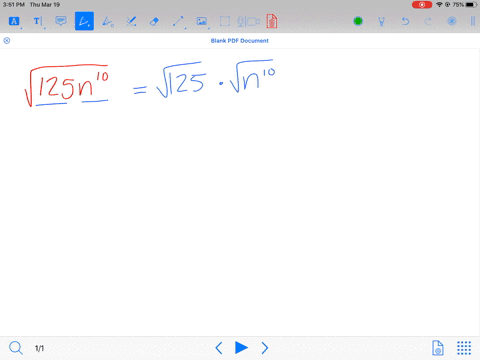Enroll in one of our FREE online STEM summer camps. Space is limited so join now!View Summer Courses### Simplify each expression. $$\sqrt{125 n^{10}}$$

01:38
KB

Need more help? Fill out this quick form to get professional live tutoring.

Get live tutoring
Problem 54

Simplify each expression.
$$\sqrt{147 k^{3}}$$

7$k \sqrt{3 k}$

## Discussion

You must be signed in to discuss.

## Video Transcript

So in this fashion we have the square root of 147 K Cube. The first thing we're gonna do is we're gonna see if we can split this up in tiny perfect squares. So 147 is the same as 49 times. Three on This is good because 49 is a perfect square. And then Kate cubed is the same. His case where a times K and this is good because Kay's a perfect square. So now we can take out a perfect square. So for Route 49 that's seven. And then for the spirit of case where that's Kay and then what's remaining under the radical A street cat. So our answer is seven K Route three K.